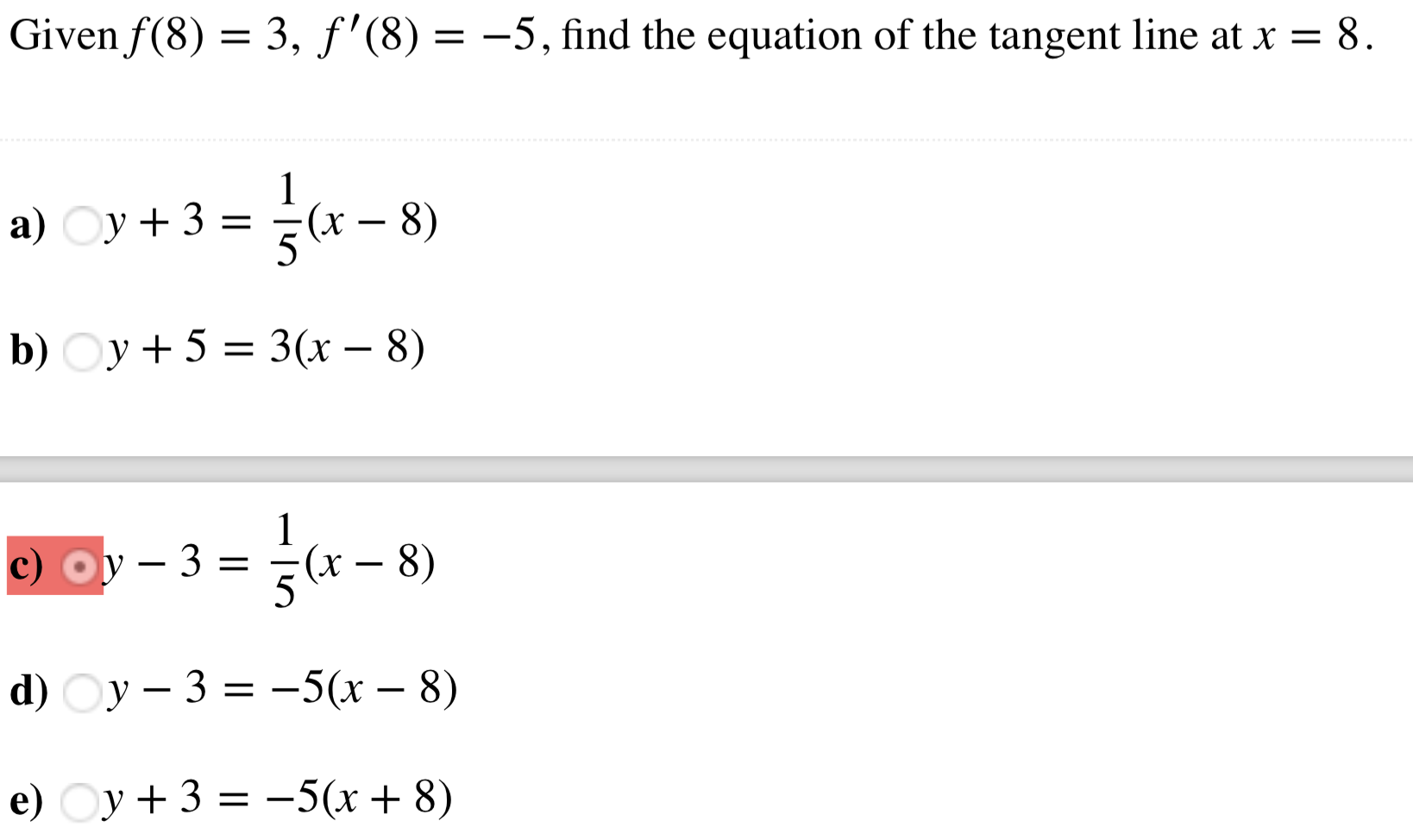Given f (8)-3, f'(8)--5, find the equation of the tangent line at x - 8a)y+3(x-8)b) Oy +5 -3(r - 8)c)3-(x - 8)

Questionhelp_outlineImage TranscriptioncloseGiven f (8)-3, f'(8)--5, find the equation of the tangent line at x - 8 a) y+3 (x-8) b) Oy +5 -3(r - 8) c) 3-(x - 8) fullscreen
Step 1

We have been given f(8) = 3 and f'(8) = -5 and we need to find the equation of tangent line x=8.

Step 2

We can use the value f(8)=3 to write the point (x1,y1) = (8,3). In addition to that, from the derivative f\'(8) = -5, we know that slope of the tangent line would be -5.

We know that general equation of a line with a known point and known slope  - aka - point slope form of line, is given as:

Step 3

Substituting the given slope and point in the point slope for...

Want to see the full answer?

See Solution

Want to see this answer and more?

Our solutions are written by experts, many with advanced degrees, and available 24/7

See Solution
Tagged in

Derivative